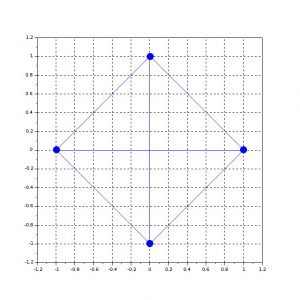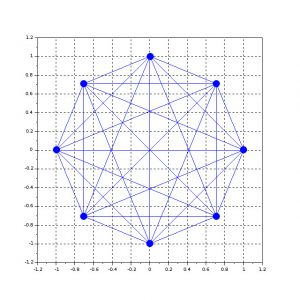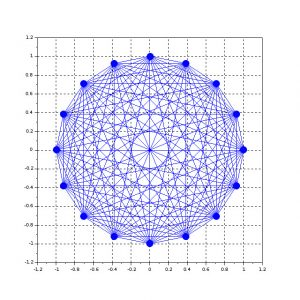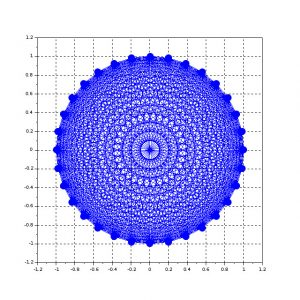# How to calculate the number of wires for a fully meshed network

Suppose we have a number of electronic control units. If we need to connect every unit with every other unit, to form a network, how many wires do we need ?

A network of electronic modules, where every module is connected with every other module, is called a fully meshed network. This kind of network topology is suitable only for small number of modules (nodes). We will see that the number of connections (wires) required in a fully meshed network grows quadratically with the number of nodes.

For example, if we have 4 nodes (electric control units) and we want to have a fully meshed network, we will need 6 wires in total.

What about for more nodes ? What is the relationship between number of nodes and number of wires ?

We have N nodes. Let’s start connecting every node with every other node. The constraints are:

• between two distinct nodes there is only one wire
• a particular node can not be connected to itself

In the table below we’re going to write down the number of connections for each node, taking into account the constraints specified above.

 Node # # of connections 1 N-1 2 N-2 … … k N-k … … N-1 1 N 0

# – number

Let’s sum up all the numbers of connections together:

$\begin{array}{lll} S_N &=& (N-1)+(N-2)+(N-3)+\dots+(N-(N-1))\\ S_N &=& (N+N+N+\dots+N) – (1+2+3+\dots+(N-1))\\ S_N &=& N(N-1) – S_N\\ 2S_N &=& N(N-1)\\ S_N &=& \frac{N(N-1)}{2}\\ \end{array}$

The number of wires SN needed to form a fully meshed network topology for N nodes is:

$\begin{equation} \begin{split} \bbox[#FFFF9D]{S_N = \frac{N(N-1)}{2}} \end{split} \end{equation}$

Example 1: N = 4

$S_N = \frac{4(4-1)}{2}=6$

Example 2: N = 8

$S_N = \frac{8(8-1)}{2}=28$

Example 3: N = 16

$S_N = \frac{16(16-1)}{2}=120$

Example 4: N = 32

$S_N = \frac{32(32-1)}{2}=496$

In the pictures below you can visualize the topology of the network for each of the above examples.Image: Fully meshed network with 4 nodesImage: Fully meshed network with 8 nodesImage: Fully meshed network with 16 nodesImage: Fully meshed network with 32 nodes

It is obvious that for networks with more than 4-6 nodes a fully meshed topology is far too expensive and complicated because of the high number of wires used. That is why, in these circumstances, a bus topology network is the right choice.

Compared to a fully meshed network, in automotive applications, a bus topology network has the following advantages:

• eliminates redundant sensors and dedicated wires for each function (lower cost, lower weight and better reliability)
• reduces the number of wires and connectors (lower cost, lower weight, better reliability, easier to package wiring harness)
• allows more features and more flexibility (modules share data, more flexible design)

For any questions, observations and queries regarding this article, use the comment form below.

Don’t forget to Like, Share and Subscribe!